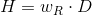## Equivalent dose

The equivalent dose is defined as the dose absorbed by body tissue through ionizing radiation, multiplied by a radiation weighting factor (see eq. 1) which accounts for the relative biological effectiveness (RBE) of the given type of radiation. As with the absorbed dose, the unit of measure for the equivalent does is J/kg as the radiation weighting factor is dimensionless. However, in order to highlight the difference to the absorbed dose, the equivalent dose received its own unit of measure, the sievert (Sv).

Eq. 1H = equivalent dose; wR = radiation weighting factor; D = absorbed dose

The natural exposure to radiation (cosmic radiation, terrestrial radiation, load of inhaled natural radon-222, intake with food) leads to a radiation dose in the range of approx. 2.1 mSv per year. Half of this value is attributed to radon inhalation alone. The dose resulting from medical applications lies in a similar range, so that a person absorbs an average of about 4 mSv per year.

In Germany, the limit value for persons exposed to radiation as part of their occupation is 20 mSv per year. A single dose of more than approx. 6 Sv is invariably fatal due to acute radiation syndrome.

Literature references:
Wikipedia